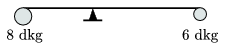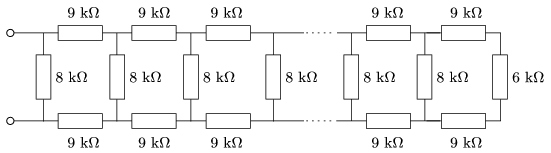Mathematical and Physical Journal
for High Schools
Issued by the MATFUND Foundation
 Already signed up? New to KöMaL?

# KöMaL Problems in Physics, May 2008

Show/hide problems of signs:## Problems with sign 'M'

Deadline expired on June 11, 2008.

M. 288. Fill a U shaped tube with water up to some height; both ends of the tube are open and the arms of the tube are vertical. Examine how the period of the oscillating water in the tube depends on the total height of the water l. Try to find the damping constant (the ratio of two consecutive amplitudes) as well.

(6 pont)

statistics## Problems with sign 'P'

Deadline expired on June 11, 2008.

P. 4074. Two students run along a circular path. While Andy runs three laps, Bob runs four laps. While they are running both of them can see only the next one-third of the path. They start running at the same time at the same starting point and they also stop running at the same time and at same position. In what fraction of the running time can they see each other?

(3 pont)

statistics

P. 4075. Can a satellite be placed above Budapest (such that it is on the line which joins the centre of the Earth and the centre of the city)?

(3 pont)

statistics

P. 4076. There is 1 litre water in a 2-l pot, the base of which has an area of 1 dm2. How much work is done if a high cylinder shaped glass of cross section 0.5 dm2 is pushed down to the bottom of the pot. The glass has negligible mass and its base is below.

(4 pont)

statistics

P. 4077. A 60-g and an 80-g ball are fixed to the ends of a 28-cm rod of negligible mass. The rod is balanced by a pivot such that it is horizontal and stays at rest. With what angular acceleration does it start to move, if the pivot is placed 2 cm closer to the heavier ball?(4 pont)

statistics

P. 4078. A hollow copper sphere is floating on the surface of water which is at a temperature of 20oC and 1% of the volume of the sphere is above the water. To what temperature must the water be heated in order to submerge the copper ball totally. (Look up how the density of water depends on the temperature of water in a data table.)

(5 pont)

statistics

P. 4079. There is a sample of neon in a cylinder which is closed by a piston. The gas is compressed such that its volume decreased to two-thirds of the original volume. The pV graph of the process is a straight line segment. In the final state the pressure of the gas is twice as much as it was originally.

a) By what factor did the absolute temperature of the gas increased?

b) How much thermal energy was absorbed by the gas from its environment?

c) How can this process be realized?

(5 pont)

statistics

P. 4080. Determine the equivalent resistance of the very long resistor sequence shown in the figure.(5 pont)

statistics

P. 4081. A neutral and a charged capacitor which have the same capacitance are connected in parallel.

a) To what fraction of the original energy does the total energy of the system decrease, after connecting the condensers?

b) What happens to the lost energy''?

c) What is the correct answer to the previous question if all the conductors are superconductors.

(5 pont)

statistics

P. 4082. Stationary'' electrons are bombarded with photons which have the same energy as the rest energy of the electrons. At most what can the maximum speed of the knocked'' electrons be?

(5 pont)

statistics

P. 4083. A 1400 year-old carbonized tree is used as sample for radiocarbon dating. The sample is contaminated by coal which does not contain radioactive carbon isotope. Will the physicist, who carries out the measurement and does not know that the sample is contaminated, find the sample older or younger than it really is? How old will the sample be determined by the physicist if it contains 30% non-radioactive coal?

(5 pont)

statistics